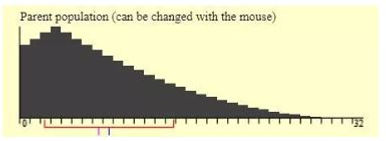## Day 97 - Lesson 6.6

##### Learning Targets
• Determine if the sampling distribution of the sample means is approximately normal when sampling from a non-normal population.

• If appropriate, use a normal distribution to calculate probabilities involving a sample mean.

##### Activity:

The goal today is for students to understand what the Central Limit Theorem does, not just to memorize it.  Using the CLT is actually very easy and we could just tell students, “Hey, if you take a sample size of 30 or more, your sampling distribution will be approximately normal.  Just memorize it.” And to be honest, we considered it.  We never want to overcomplicate something just for the sake of doing an activity, but the Central Limit Theorem is essentially what allows to do statistics for the rest of the year.  It’s too important.  Our consciences wouldn’t let us tell the kids just to memorize it.  We wanted them to see it at work.

So we used an applet to help us with that.  The context for today is comparing out school ACT scores to the ACT scores of an area rival school.  You should definitely change the school names to reflect your school and rival.  We set up the applet so that the ACT scores at our school are approximately normal with a mean of 16.  As students begin taking samples in the applet, they should see the sampling distribution is approximately normal.  This is what we learned in lesson 6.5.But what happens if the population is not approximately normal?  That’s where the rival school comes in.  We’ve set their population to be skewed right with a mean of 8.08 (which is of course ridiculous but, hey, what are rivalries for?).Students will take samples of size 2 and 16.  After taking many samples they should see that the sampling distribution when n = 2 is still skewed right while the sampling distribution when n = 16 is becoming more normal.

Ask students how we could try to get the sampling distribution even closer to normal.  Finally, after this discussion, we give them the specifics of the Central Limit Theorem.The activity is pretty straight forward.  You may want to model the first couple of samples for your students.  Make sure that all options and menus are exactly as described in the activity.